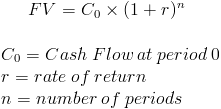Custom Search

# Future Value

Future Value Calculator (Click Here or Scroll Down)Future Value (FV) is a formula used in finance to calculate the value of a cash flow at a later date than originally received. This idea that an amount today is worth a different amount than at a future time is based on the time value of money.

The time value of money is the concept that an amount received earlier is worth more than if the same amount is received at a later time. For example, if one was offered \$100 today or \$100 five years from now, the idea is that it is better to receive this amount today. The opportunity cost for not having this amount in an investment or savings is quantified using the future value formula. If one wanted to determine what amount they would like to receive one year from now in lieu of receiving \$100 today, the individual would use the future value formula. See example at the bottom of the page.

The future value formula also looks at the effect of compounding. Earning .5% per month is not the same as earning 6% per year, assuming that the monthly earnings are reinvested. As the months continue along, the next month's earnings will make additional monies on the earnings from the prior months. For example, if one earns interest of \$40 in month one, the next month will earn interest on the original balance plus the \$40 from the previous month. This is known as compound interest.

### Use of Future Value

The future value formula is used in essentially all areas of finance. In many circumstances, the future value formula is incorporated into other formulas. As one example, an annuity in the form of regular deposits in an interest account would be the sum of the future value of each deposit. Banking, investments, corporate finance all may use the future value formula is some fashion.

#### Example of Future Value Formula

An individual would like to determine their ending balance after one year on an account that earns .5% per month and is compounded monthly. The original balance on the account is \$1000. For this example, the original balance, which can also be referred to as initial cash flow or present value, would be \$1000, r would be .005(.5%), and n would be 12 (months).

Putting this into the formula, we would have:After solving, the ending balance after 12 months would be \$1061.68.

As a side note, notice that 6% of \$1000 is \$60. The additional \$1.68 earned in this example is due to compounding.

##### Alternative Formula

The Future Value formula may also be shown asNew to Finance?